# 3-Decomposition Conjecture

 Importance: High ✭✭✭
 Author(s): Arthur Hoffmann-Ostenhof
 Subject: Graph Theory
 Keywords: cubic graph
 Posted by: arthur on: January 24th, 2017
Conjecture   (3-Decomposition Conjecture) Every connected cubic graphhas a decomposition into a spanning tree, a family of cycles and a matching.

We state the conjecture in a more precise manner:

Letbe a connected cubic graph. Thencontains a spanning tree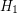, a-regular subgraphand a matching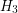(where onlyand notormay be empty) such thatand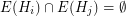for everywith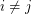.

The conjecture holds for all hamiltionian cubic graphs and for all connected planar cubic graphs, see  and see also .

Every cubic graph G which has a spanning tree T such that every vertex of T has degree three or one (such spanning tree T is called a HIST) obviously satisfies this conjecture. But not every connected cubic graph has a HIST, see .

The 3-Decomposition Conjecture has been shown to be equivalent to the following conjecture:

Conjecture  (2-Decomposition Conjecture) Letbe connected graph where every vertex has degree two or three. Suppose that for every cycleof,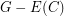is disconnected, thenhas a decomposition into a spanning treeand a matching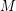, i.e.

Note that every cyclewhich passes through a vertex of degree two satisfies the condition that G-E(C) is disconnected.

Remark: The 3-Decomposition Conjecture has also been shown to hold for other classes of cubic graphs, see for instance [3,4]. A survey on the 3-Decompostion conjecture has been given by the author 2015 in Pilsen (at that time the planar case was still open) see iti.zcu.cz/plzen15/talks/1-2a-Arthur-Survey_decomposition.ppt (and press play if you find the play button). Note that there are several papers on the problem whether a planar graphhas a matchingsuch that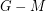is acyclic, see for instance .

## Bibliography

 Arthur Hoffmann-Ostenhof, Tomáš Kaiser, Kenta Ozeki, \arXiv[Decomposing planar cubic graphs] 1609.05059 [math.CO]
 Arthur Hoffmann-Ostenhof, Kenta Ozeki, \arXiv[On HISTs in Cubic Graphs] 1507.07689 [math.CO]
 F. Abdolhosseini, S. Akbari, H. Hashemi, M.S. Moradian, \arXiv[Hoffmann-Ostenhof's conjecture for traceable cubic graphs] 1607.04768[math.CO]
 Anna Bachstein, Dong Ye (talk): www.rwoodroofe.math.msstate.edu/workshop2014/bachstein_slides.pdf
 Arthur Hoffmann-Ostenhof (talk): www.iti.zcu.cz/plzen15/talks/1-2a-Arthur-Survey_decomposition.ppt
 Yingqian Wang, Qijun Zhang, Discrete Mathematics 311 (2011) 844–849, Decomposing a planar graph with girth at least 8 into a forest and a matching
 Kenta Ozeki, Dong Ye, Decomposing plane cubic graphs, European Journal of Combinatorics 52 (2016) 40-46.

* indicates original appearance(s) of problem.